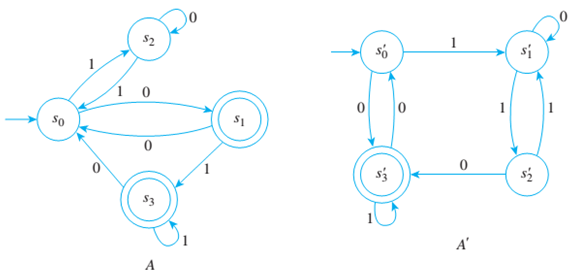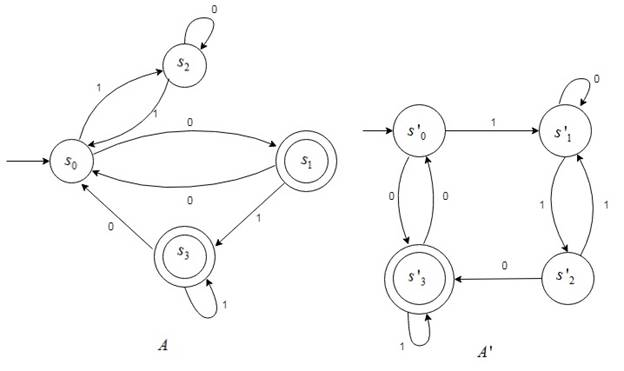Chapter 12.3, Problem 7ES### Discrete Mathematics With Applicat...

5th Edition
EPP + 1 other
ISBN: 9781337694193

#### Solutions

Chapter
Section### Discrete Mathematics With Applicat...

5th Edition
EPP + 1 other
ISBN: 9781337694193
Textbook Problem
1 views

# Are the automata A and A’ shown below equivalent?To determine

Are the automata Aand A' shown below equivalent?Explanation

Given information:

Concept used:

It is required to show that the automata shown above are equivalent. If the quotient transition diagram of the two automata are same then the two automata are equivalent.

Calculation:

For A :

0 Equivalence classes- Two states are zero equivalent if both are accepting states or both are

non-accepting states. Therefore, the 0 equivalence classes are: {s0,s2},{s1,s3}

1 Equivalence classes- Two states are 1 equivalent if and only if they are 0 equivalent and after input of any symbol, their next state are 0 equivalent. Therefore, the 1 equivalence classes are: {s0},{s2},{s1,s3}

2 Equivalence classes- Two states are called 2 equivalent if and only if they are 1 equivalent and after input of any symbol, their next state are 1 equivalent. Therefore, the 2 equivalent classes are: {s0},{s2},{s1,s3}

The transition diagram for the quotient automaton A¯ is

For A' :

0 Equivalence classes- Two states are zero equivalent if both are accepting states or both are

non-accepting states

### Still sussing out bartleby?

Check out a sample textbook solution.

See a sample solution

#### The Solution to Your Study Problems

Bartleby provides explanations to thousands of textbook problems written by our experts, many with advanced degrees!

Get Started